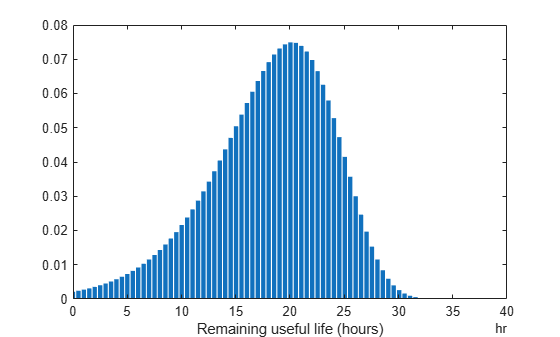# reliabilitySurvivalModel

Probabilistic failure-time model for estimating remaining useful life

## Description

Use `reliabilitySurvivalModel` to estimate the remaining useful life (RUL) of a component using a probability distribution of component failure times. Reliability survival models are useful when the only data you have are the failure times for an ensemble of similar components, such as multiple machines manufactured to the same specifications.

To configure a `reliabilitySurvivalModel` object for a specific type of component, use `fit`, which estimates the probability distribution coefficients from a collection of failure-time data. Once you configure the parameters of your reliability survival model, you can then predict the remaining useful life of similar components using `predictRUL`. For a basic example illustrating RUL prediction, see Update RUL Prediction as Data Arrives.

For general information on predicting remaining useful life, see Models for Predicting Remaining Useful Life.

## Creation

### Syntax

``mdl = reliabilitySurvivalModel``
``mdl = reliabilitySurvivalModel(distribution)``
``mdl = reliabilitySurvivalModel(initModel)``
``mdl = reliabilitySurvivalModel(___,Name,Value)``

### Description

example

````mdl = reliabilitySurvivalModel` creates a reliability survival model for estimating RUL model that uses a Weibull distribution and initializes the model with default settings.```
````mdl = reliabilitySurvivalModel(distribution)` creates a reliability survival model that uses the specified probability distribution function and sets the `Distribution` property of the model.```
````mdl = reliabilitySurvivalModel(initModel)` creates a reliability survival model and initializes the model parameters using an existing `reliabilitySurvivalModel` object `initModel`.```

example

````mdl = reliabilitySurvivalModel(___,Name,Value)` specifies user-settable model properties using name-value pairs. For example, `reliabilitySurvivalModel('LifeTimeUnit',"days")` creates a reliability survival model that uses days as a lifetime unit. You can specify multiple name-value pairs. Enclose each property name in quotes.```

### Input Arguments

expand all

Reliability survival model, specified as a `reliabilitySurvivalModel` object.

## Properties

expand all

Probability distribution function used to model the lifetime distribution, specified as one of the following:

Distribution StringDistribution Object
`"BirnbaumSaunders"``BirnbaumSaundersDistribution`
`"Exponential"``ExponentialDistribution`
`"Gamma"``GammaDistribution`
`"GeneralizedPareto"``GeneralizedParetoDistribution`
`"HalfNormal"``HalfNormalDistribution`
`"InverseGaussian"``InverseGaussianDistribution`
`"Kernel"``KernelDistribution`
`"Logistic"``LogisticDistribution`
`"Loglogistic"``LoglogisticDistribution`
`"Lognormal"``LognormalDistribution`
`"Nakagami"``NakagamiDistribution`
`"Normal"``NormalDistribution`
`"Poisson"``PoissonDistribution`
`"Rayleigh"``RayleighDistribution`
`"Stable"``StableDistribution`
`"Weibull"``WeibullDistribution`

To configure the parameters of the probability distribution function, use the `fit` function.

Distribution coefficients estimated by the `fit` function, specified as a vector. For more information on the coefficients of each distribution function, see the corresponding distribution object listed in `Distribution`. For more information on model fitting, see `fitdist`.

Covariance of the distribution coefficients estimated by the `fit` function, specified as a positive array with size equal to the number of coefficients. For more information on the coefficients of each distribution function, see the corresponding distribution object listed in `Distribution`.

Distribution coefficient names assigned when the model is trained using the `fit` function, specified as string array. For more information on the coefficients of each distribution function, see the corresponding distribution object listed in `Distribution`.

Censor variable, specified as a string that contains a valid MATLAB® variable name. The censor variable is a binary variable that indicates which life-time measurements in `data` are not end-of-life values.

`CensorVariable` must not match any of the strings in `DataVariables` or `LifeTimeVariable`.

You can specify `CensorVariable`:

• Using a name-value pair when you create the model

• As an argument when you call the `fit` function

• Using dot notation after model creation

Lifetime variable, specified as a string that contains a valid MATLAB variable name. For survival models, the lifetime variable contains the historical life span measurements of components.

You can specify `LifeTimeVariable`:

• Using a name-value pair when you create the model

• As an argument when you call the `fit` function

• Manually using dot notation

Lifetime variable units, specified as a string.

The units of the lifetime variable do not need to be time-based. The life of the test component can be measured in terms of a usage variable, such as distance traveled (miles) or fuel consumed (gallons).

Data variables, specified as an empty string. This property is ignored for reliability survival models.

Additional model information for bookkeeping purposes, specified as any data type or format. The model does not use this information.

You can specify `UserData`:

• Using a name-value pair when you create the model

• Using dot notation after model creation

## Object Functions

 `predictRUL` Estimate remaining useful life for a test component `fit` Estimate parameters of remaining useful life model using historical data

## Examples

collapse all

`load('reliabilityData.mat')`

This data is a column vector of `duration` objects representing battery discharge times.

Create a reliability survival model with default settings.

`mdl = reliabilitySurvivalModel;`

Train the survival model using the training data.

`fit(mdl,reliabilityData,"hours")`

`load('reliabilityData.mat')`

This data is a column vector of `duration` objects representing battery discharge times.

Create a reliability survival model, specifying the life time variable and life time units.

`mdl = reliabilitySurvivalModel('LifeTimeVariable',"DischargeTime",'LifeTimeUnit',"hours");`

Train the survival model using the training data.

`fit(mdl,reliabilityData)`

Predict the life span of a new component, and obtain the probability distribution function for the estimate.

`[estRUL,ciRUL,pdfRUL] = predictRUL(mdl);`

Plot the probability distribution.

```bar(pdfRUL.RUL,pdfRUL.ProbabilityDensity) xlabel('Remaining useful life (hours)') xlim(hours([40 90]))```Improve the distribution view by providing the number of bins and bin size for the prediction.

```[estRUL,ciRUL,pdfRUL] = predictRUL(mdl,'BinSize',0.5,'NumBins',500); bar(pdfRUL.RUL,pdfRUL.ProbabilityDensity) xlabel('Remaining useful life (hours)') xlim(hours([40 90]))```Predict the RUL for a component that has been operating for 50 hours.

```[estRUL,ciRUL,pdfRUL] = predictRUL(mdl,hours(50),'BinSize',0.5,'NumBins',500); bar(pdfRUL.RUL,pdfRUL.ProbabilityDensity) xlabel('Remaining useful life (hours)') xlim(hours([0 40]))```## Version History

Introduced in R2018a